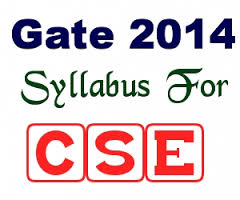## GATE Syllabus for Computer Science (CSE) and IT 2014 PDF Download

GATE Syllabus for Computer Science (CSE) and IT 2014 PDF Download : 1. Computer Science (CS) stream is provided  by almost all the engineering colleges, so in order to gain better knowledge in this field candidates plan for their masters.2. M.Tech is one of the post graduation course which is taken by most of the Computer Science (CS) students. All these candidates must appear for GATE exam to get admission in any college.

3. Look at the syllabus for Computer Science (CS) in GATE-2014 exam

4. ENGINEERING MATHEMATICS
Mathematical Logic : Propositional Logic; First Order Logic.

Probability : Conditional Probability; Mean, Median, Mode and Standard Deviation; Random Variables; Distributions; uniform, normal, exponential, Poisson, Binomial.

Set Theory & Algebra : Sets; Relations; Functions; Groups; Partial Orders; Lattice; Boolean Algebra.

Combinatory : Permutations; Combinations; Counting; Summation; generating functions; recurrence relations; asymptotics.

Graph Theory : Connectivity; spanning trees; Cut vertices & edges; covering; matching; independent sets; Colouring; Planarity; Isomorphism.

Linear Algebra : Algebra of matrices, determinants, systems of linear equations, Eigen values and Eigen vectors.

Numerical Methods : LU decomposition for systems of linear equations; numerical solutions of non-linear algebraic equations by Secant, Bisection and Newton-Raphson Methods; Numerical integration by trapezoidal and Simpson’s rules.

Calculus : Limit, Continuity & differentiability, Mean value Theorems, Theorems of integral calculus, evaluation of definite & improper integrals, Partial derivatives, Total derivatives, maxima & minima.

5. COMPUTER SCIENCE AND INFORMATION TECHNOLOGY :

Digital Logic : Logic functions, Minimization, Design and synthesis of combinational and sequential circuits; Number representation and computer arithmetic (fixed and floating point).

Computer Organization and Architecture : Machine instructions and addressing modes, ALU and data-path, CPU control design, Memory interface, I/O interface (Interrupt and DMA mode), Instruction pipelining, Cache and main memory, Secondary storage.

Programming and Data Structures : Programming in C; Functions, Recursion, Parameter passing, Scope, Binding; Abstract data types, Arrays, Stacks, Queues, Linked Lists, Trees, Binary search trees, Binary heaps.

Algorithms : Analysis, Asymptotic notation, Notions of space and time complexity, Worst and average case analysis; Design: Greedy approach, Dynamic programming, Divide-and-conquer; Tree and graph traversals, Connected components, Spanning trees, Shortest paths; Hashing, Sorting, Searching. Asymptotic analysis (best, worst, average cases) of time and space, upper and lower bounds, Basic concepts of complexity classes – P, NP, NP-hard, NP-complete.

Theory of Computation : Regular languages and finite automata, Context free languages and Push-down automata, Recursively enumerable sets and Turing machines, Undecidability.

Compiler Design : Lexical analysis, Parsing, Syntax directed translation, Runtime environments, Intermediate and target code generation, Basics of code optimization.

Operating System : Processes, Threads, Inter-process communication, Concurrency, Synchronization, Deadlock, CPU scheduling, Memory management and virtual memory, File systems, I/O systems, Protection and security.

Databases : ER-model, Relational model (relational algebra, tuple calculus), Database design (integrity constraints, normal forms), Query languages (SQL), File structures (sequential files, indexing, B and B+ trees), Transactions and concurrency control.

Information Systems and Software Engineering : information gathering, requirement and feasibility analysis, data flow diagrams, process specifications, input/output design, process life cycle, planning and managing the project, design, coding, testing, implementation, maintenance.

Computer Networks : ISO/OSI stack, LAN technologies (Ethernet, Token ring), Flow and error control techniques, Routing algorithms, Congestion control, TCP/UDP and sockets, IP(v4), Application layer protocols (icmp, dns, smtp, pop, ftp, http); Basic concepts of hubs, switches, gateways, and routers. Network security – basic concepts of public key and private key cryptography, digital signature, firewalls.

Web technologies : HTML, XML, basic concepts of client-server computing.

6. Visit the official website of GATE-2014 for more details -  http://gate.iitkgp.ac.in/gate2014/

7. Candidates join us on Facebook to receive latest news and updates about GATE 2014 syllabus, GATE 2014 admit card, GATE 2014 exam date, GATE 2014 results, GATE 2014 counselling, GATE 2014 sample papers. Guys bookmark our page or subscribe us for further reference.